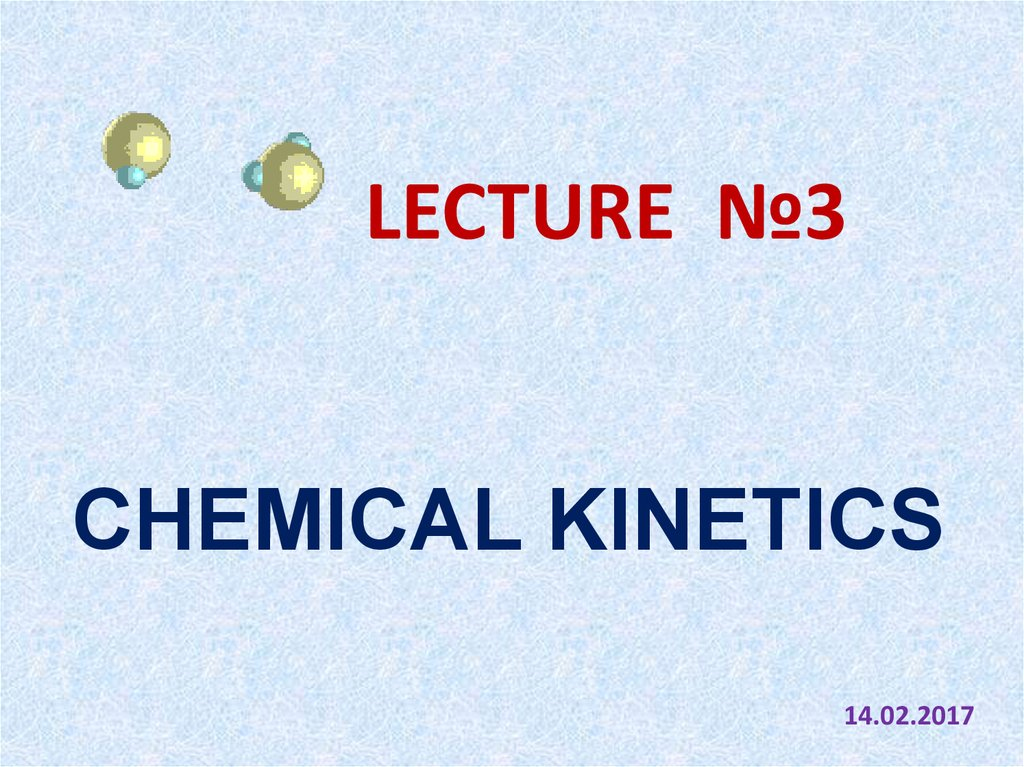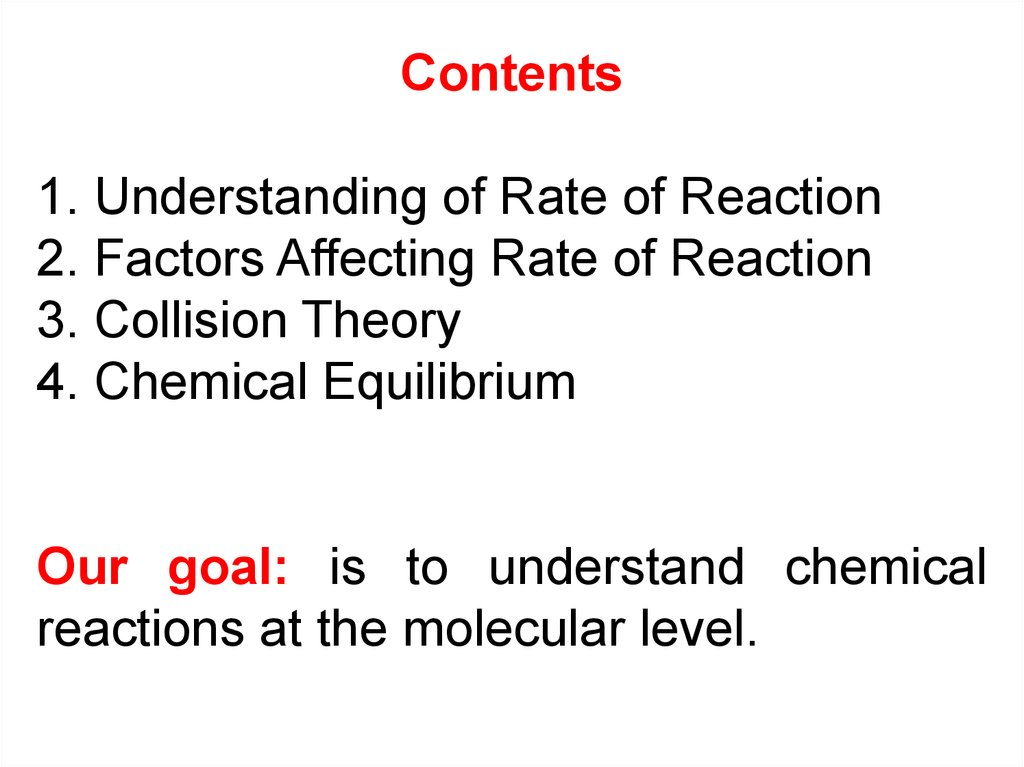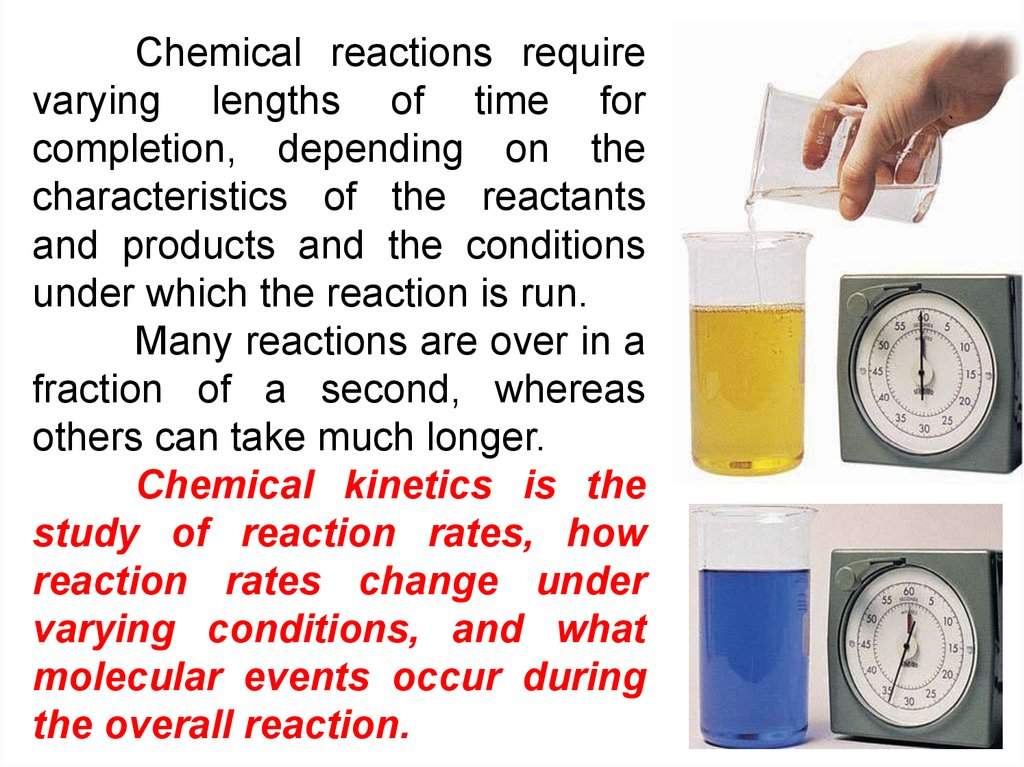Chemical kinetics

1.

LECTURE №3
CHEMICAL KINETICS
14.02.2017

2.

Contents
1. Understanding of Rate of Reaction
2. Factors Affecting Rate of Reaction
3. Collision Theory
4. Chemical Equilibrium
Our goal: is to understand chemical
reactions at the molecular level.

3.

Chemical reactions require
varying lengths of time for
completion, depending on the
characteristics of the reactants
and products and the conditions
under which the reaction is run.
Many reactions are over in a
fraction of a second, whereas
others can take much longer.
Chemical kinetics is the
study of reaction rates, how
reaction rates change under
varying conditions, and what
molecular events occur during
the overall reaction.

4.

Chemical kinetics is the study of the speed with which
a chemical reaction occurs and the factors that affect this
speed. This information is especially useful for determining
how a reaction occurs.
The rate of a reaction is the amount of product formed or
the amount of reactant used up per unit of time.
Therefore, the reaction rate is the increase in molar
concentration of product of a reaction per unit time or
the decrease in molar concentration of reactant per unit
time. The usual unit of reaction rate is moles per liter
per second, mol/l*s.
2
1
C C
2
1
C

5.

During chemical reaction, reactants are
consumed, products are formed.
Amount of reactants decreases↓, amount of
product increases↑
A gas
B gas

6.

THE RATE OF CHEMICAL REACTION
HOMOGENEUOS
REACTION RATE
is the change moles of
reactants in volume (l) of
solution per unit time
HETEROGENEUOS
REACTION RATE
is the change moles
reactants on 1m2 surface
per unit time
n( X )
S t
n( X )
V t
mol/м3 s
mol/м2 s
mol/l s

7.

FACTORS AFFECTING THE REACTION
RATE:
• Nature of the reactant (gas, liquid or
solid)
• Surface area
• Concentration
• Temperature
• Catalyst
• Pressure (gases)

8.

Nature of the reactant and products
In organic reaction a large number of bonds
are broken and a large number of bonds are
formed. Such reactions proceed at a slower
rate: C H O H O C H O C H O
12 22 11
2
6 12 6
6 12 6
sugar
glucose
fructose
In ionic reactions the reactants are in ionic
form and no bonds have to be broken up. Such
reactions proceed at a faster rate:
Ag NO Na Cl AgCl Na NO
3
3
cation
anion

9.

HOW SURFACE AREA AFFECTS THE RATE OF
REACTION?
CaCO3(s)+2HCl(ℓ) → CaCl2(s)+H2O(ℓ)+CO2(g)
marble piece
marble powder
HCl dilute

10.

LAW OF MASS ACTION
At constant temperature, the rate of a chemical
reaction is directly proportional to the product of the
molar concentrations of reacting species, with each
concentration term raised to the power equal to the
numerical coefficient of the species in the chemical
equilibrium:
aA + bB сС + dD
k [ A] [ B]
a
b
here k is the reaction rate constant that depends on
temperature:
• It has a fixed value at a particular temperature.
• Value of K varies with temperature.
• Value of K remains unaltered with the change in concentration of
reactants.

11.

FOR EXAMPLE:
Homogeneous reactions which occur in one
phase only. It may be a gaseous phase or a liquid
phase:
2SO2(g) + O2(g) = 2SO3(g)
Rate of forward reaction: 1= k1 ·[SO2] 2·[O2]
Rate of backward reaction: 2=k2 ·[SO3] 2
Heterogeneous reactions which take place in
two or more phases (e.g., gaseous reactions taking
place on the surface of a solid catalyst or on the walls
of the container):
4Al(s) + 3O2(g) = 2Al2O3(s)
1= k1 ·4·[O2] 3 = k1·[O2] 3
2=k2 · 2 = k2

12.

THE EFFECT OF TEMPERATURE ON REACTION RATE
described by principle of Vant Hoff “In the temperature
range from 0 C to 100 C, at a temperature increase of 10 C
degrees the rate of chemical reaction increases by 2 – 4
times”
- temperature coefficient is a number indicating how
many times will increase the reaction rate with increasing
temperature at 10 C
An example: how to change the speed
t 2 t1
of the reaction at a temperature
10
increase of 40 C, if the temperature
t2
t1
coefficient is equal to 3:
t
40
t 2 t1
4
10
10
t2
10
V V
V
Vt1
V 3 3 81
The reaction rate increases 81-fold

13.

Why does increased temperature increase the rate of reaction?
At a higher temperature, particles have more energy. This means they
move faster and are more likely to collide with other particles. When the particles
collide, they do so with more energy, and so the number of successful collisions
increases.
Collision theory states that before any chemical reactions can occur,
particles of the reactants have to collide with each other .
Effective collision – collision that produces chemical reactions by achieving
the minimum energy and correct collision orientation .
Activation energy is the minimum energy needed by the reactant particles to
react and it is different for each reation.
Colliding particles must have equal or be more than the activation energy.
I
H
H
I
I
Correct collision orientation
H
I
H
H
I
H
Effective collision
I
Reaction happens

14.

The rate of a reaction depends on the temperature at
which it is run. As the temperature increases, the molecules
move faster and therefore collide more frequently. The
molecules also carry more kinetic energy. Thus, the proportion
of collisions that can overcome the activation energy for the
reaction increases with temperature.
The only way to explain the relationship between
temperature and the rate of a reaction is to assume that the
rate constant depends on the temperature at which the
reaction is run. In 1889, Svante Arrhenius showed that the
relationship between temperature and the rate constant
for a reaction obeyed the following equation:
Ea
k A exp (
)
RT
A is the proportionality
constant called the
frequency factor or pre
exponential factor.

15. DETERMINE THE ACTIVATION ENERGY

Ea
k A exp ( )
RT
Ea
ln k ln A
RT
R T1 T2
k1 2,3 R T1 T2 k 2
Ea
ln
lg
T1 T2
k2
T2 T1
k1
Еа
> 100 kJ – big value, the reaction proceeds very
slowly
Еа < 50 kJ – small value, the reaction proceeds very
quickly

16.

CHEMICAL EQUILIBRIUM
The irreversible reaction moves in one
direction only from reactant to product:
t
2KClO3 2KCl 3O2
The reversible reactions can move in both
directions. The stage of reversible reaction when
the rate of forward reaction becomes equal to rate
of back reaction is known as equilibrium and can
be represented in terms of equilibrium constant.
N 2( g ) 3H 2( g ) 2 NH 3( g )

17.

aA + bB сС + dD
Forward reaction:
Back reaction:
1 k1 [ A] [ B]
a
2 k 2 [C ] [ D]
c
d
[C] [ D]
KP
a
b
[
A
]
[
B
]
k2
k1
Equilibrium constant:
b
c
d
"At a given temperature, the product of concentrations of the
reaction product each raised to the respective stoichiometric
coefficients in the balanced chemical equation divided by the
product of concentrations of the reactants raised to their individual
stoichiometric coefficients has a constant value“

18.

Value of chemical equilibrium constant
0
No
Reversible
reaction
occurs 10-6 reaction 106
Irreversible
reaction

19.

N 2( g ) 3H 2( g ) 2 NH 3( g )
1 k1 [ N 2 ] [ H 2 ]3
HETEROGENEOUS
REACTION
2 k 2 [ NH 3 ] 2
1 2
2
[ NH 3 ]
KP
[ N 2 ] [ H 2 ]3
2 Na( S ) 2 H 2 O( aq) 2 NaOH ( aq) H 2
1 k1 [ H 2 O ] 2
2 k 2 [ NaOH ] 2 [ H 2 ]
1 2
HOMOGENEOUS
REACTION
[ NaOH ] 2 [ H 2 ]
KP
[ H 2 O]2
(g)

20.

The most important condition for the
equilibrium is that ΔG of the reaction should be
0.
G = 0
The reaction is at equilibrium
This means when ΔG is zero both the
forward reaction and reverse reaction takes
place simultaneously.
0
G
= – RT lnKP

21.

CONDITIONS THAT AFFECT THE EQUILIBRIUM
The conditions that affect the equilibrium are
described according to Le Chatelier’s principle:
Анри-Луи Ле-Шателье
(1850–1936 )
If a system at equilibrium is disturbed by changing
the variables such as pressure, temperature or
concentration, then the system will tend to adjust
itself so as to minimize the effect of that change as
far as possible.
This principle of Le Chatelier’s highlights the behavior of
a system at equilibrium, if it is subjected to changes in
parameters like pressure, temperature, or concentration.

22.

FACTORS AFFECTING EQUILIBRIUM
Some of the factors which affect the
equilibrium constant value are:
1. Concentration. If the concentration of
one ingredient is changed, the equilibrium
shifts toward the formation of products, i.e. in
the direction of the reaction which occurs with
the greatest speed and reduces the
concentration of the reactants.
2. Temperature. Change in temperature
shifts the equilibrium to the favorable side, i.e.
towards the endothermic reaction, and vice
versa.

23.

3. Pressure. The pressure change affects
only on those systems where in at least one of
the substances in the gaseous state. As the
pressure increases, the equilibrium shifts in the
direction of decreasing the amount of gaseous
substances, i.e. towards of fewer moles of
gaseous substances, and vice versa.
4. Catalyst does not affect to
Equilibrium, it may increases both the forward
as well as reverse reaction speeds.

24.

CATALYST
• A catalyst will change the rate of reaction.
• A catalyst only changes the rate of reaction but not the
quantity of products.
• A catalyst does not undergo any chemical change at the
end of the reaction.
PRESSURE
• Pressure can affect the rate of reaction only if it
involves gases.
• A higher pressure can increase the rate of reaction.
• The increase of pressure will compress the gas.
• The particles of a gas will collide more frequently when
in a compressed state (smaller volume).

25.

Catalysts are substances that change the rate of a
reaction without itself being consumed.
Catalysts never produce more product – they just
produce the same amount more quickly.
Catalysts increase the rate of a reaction by decreasing
the activation energy of the reaction.
Catalysts change the mechanism by which the process
occurs.
ratecatalyzed > rateuncatalyzed

26. QUIZ ME

1
What is the discipline that studies chemical
reactions with respect to reaction rates, effect of
various variables, rearrangement of atoms,
formation of intermediates etc.?
Electrochemistry
Chemical thermodynamics
Physical thermodynamics
Chemical kinetics
NEXT

27. QUIZ ME

2
What drives chemical reactions?
Energy
Activation Energy
Electrons
Physical conditions
NEXT

28. QUIZ ME

3
Which one of the following reactions reacts
the most rapidly at room temperature??
2 H2 + O2 -> 2 H2O
C12H22O11 (sugar) -> 12 C + 11 H2O
H+ + OH- -> H2O (neutralization)
*H + OH -> H2O (radical reaction)
NEXT